A Technical Method For Rating Stocks

The WinWayCharts EDScode based on Markos Katsanos’ article in this issue, “A Technical Method For Rating Stocks,” is shown below.
Synopsis from Stocks & Commodities June 2018
I’s it possible to create a stock rating system using multiple indicators or other technical criteria? If so, how does it compare with analyst ratings? Investors around the world move billions of dollars every day on advice from Wall Street research analysts. Most retail investors do not have the time or can’t be bothered to read the full stock report and rely solely on the bottom line: the stock rating. They believe these ratings are reliable and base their investment decisions at least partly on the analyst buy/sell rating. But how reliable are those buy/sell ratings? In this article I will present a technical stock rating system based on five technical criteria and indicators, backtest it, and compare its performance to analyst ratings.
```!A TECHNICAL METHOD FOR RATING STOCKS
!Author: Markos Katsanos, TASC June 2018
!Coded by: Richard Denning, 4/18/18

!INPUTS:
MAP is 63.
STIFFMAX is 7.
VFIPeriod is 130.
MASPY is 100.
SCORECRIT is 5.
W1 is 1.
W2 is 1.
W3 is 1.
W4 is 1.
W5 is 2.

!VFI FORMULA:
COEF is 0.2.
VCOEF is 2.5.
Avg is ([high]+[low]+[close])/3.
inter is ln( Avg ) - ln( Valresult( Avg, 1 ) ).
vinter is sqrt(variance(inter, 30 )).
cutoff is Coef * Vinter * [Close].
vave is Valresult(simpleavg([volume], VFIPeriod ), 1 ).
vmax is Vave * Vcoef.
vc is Min( [volume], VMax ).
mf is Avg - Valresult( Avg, 1 ).
vcp is iff(MF > Cutoff,VC,iff(MF < -Cutoff,-VC,0)).
vfitemp is Sum(VCP , VFIPeriod ) / Vave.
vfi is expavg(VFItemp, 3 ).

!STIFFNESS
ma100 is Avg.
CLMA if [close] < MA100.
STIFFNESS is countof(CLMA,MAP).

!CONDITIONS:
! MONEY FLOW:
COND1 is iff(VFI>0,1,0).
!SIMPLEAVG:
COND2 is iff([close]>SMA,1,0).
!SIMPLEAVG DIRECTION:
COND3 is iff(SMA>valresult(SMA,4),1,0).
!STIFFNESS:
COND4 is iff(STIFFNESS<= STIFFMAX,1,0).
!MARKET DIRECTION:
SPY is TickerUDF("SPY",[close]).
COND5 is iff(EXPAVG(SPY,MASPY)>=
valresult(EXPAVG(SPY,MASPY),2),1,0).

SCORE is  W1*COND1+W2*COND2+W3*COND3+
W4*COND4+W5*COND5.

```
Figure 11 shows the summary results of a backtest using NASDAQ 100 stocks during a generally bullish period from April 2009 to April 2018. Figure 12 shows the backtest using the same list of NASDAQ 100 stocks during a period that had two bear markets (April 1999 to April 2009). The average results are similar except that there are fewer trades during the period that contained the two bear markets. Both backtests use a fixed 21-bar exit.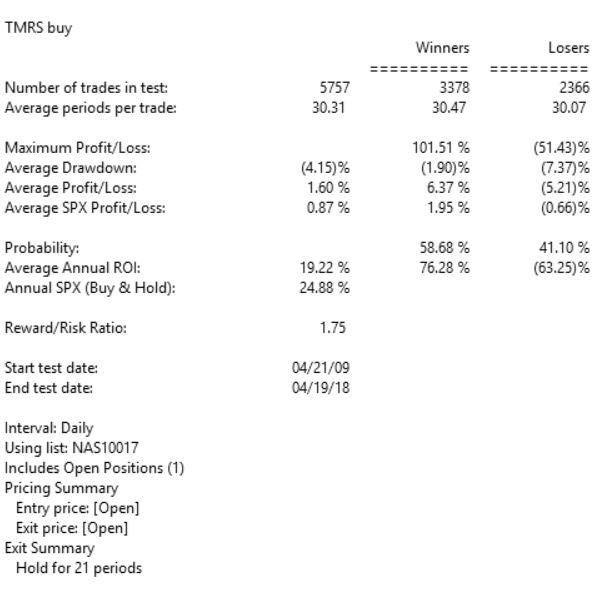FIGURE 11: AIQ, BULL MARKET. Here are the summary results of a backtest using NASDAQ 100 stocks during a generally bullish period from April 2009 to April 2018.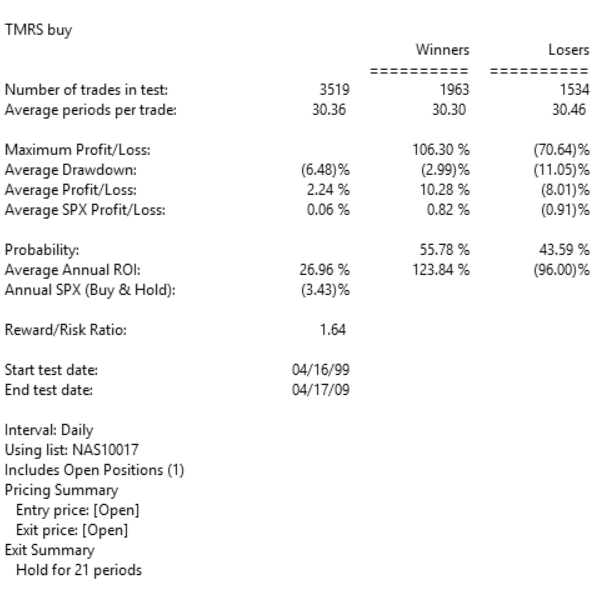FIGURE 12: AIQ, BEAR MARKET. Here are the summary results of a backtest using NASDAQ 100 stocks during a period from April 1999 to April 2009 that contained two bear markets.
—Richard Denning info@TradersEdgeSystems.com for AIQ Systems

Weekly and Daily MACD

The WinWay EDS code based on Vitali Apirine’s article in December 2017 issue of Stocks and Commodities magazine, “Weekly & Daily MACD,” is provided below.
The moving average convergence/divergence oscillator (MACD), developed by Gerald Appel, is one of the more popular technical analysis indicators. The MACD is typically used on a single timeframe, but what if we looked at two timeframes on one chart?

Traders can look for relative daily MACD line crossovers, weekly and daily centerline crossovers, and divergences to generate trading signals.
Figure 5 shows the daily & weekly MACD indicator on a chart of Apple Inc. (AAPL) during 2016 and 2017, when there was a change from a downtrend to an uptrend.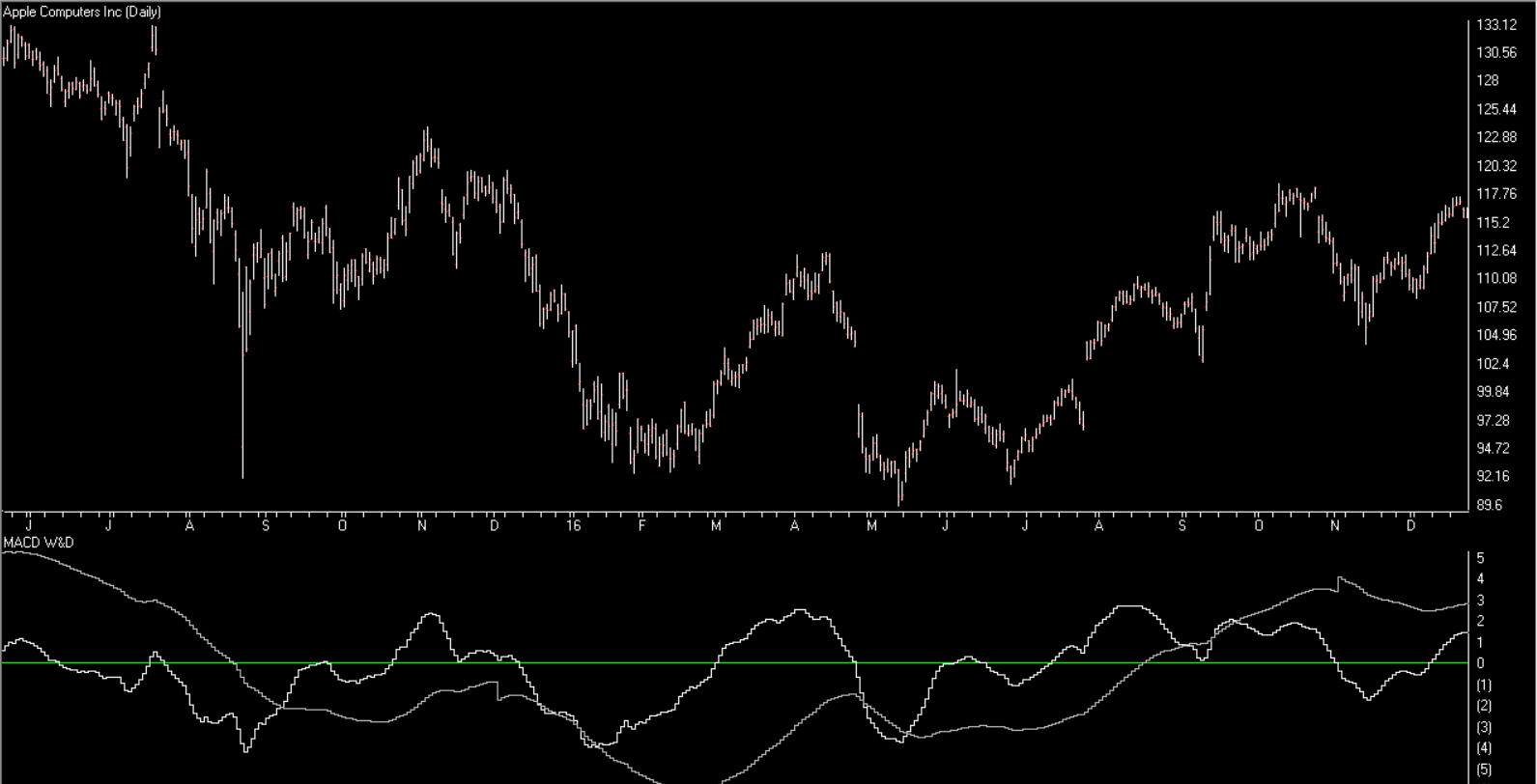FIGURE 5: AIQ. Here is an example of the daily & weekly MACD on a chart of AAPL.
The code and EDS file can be downloaded from http://aiqsystems.com/dailyweeklyMACD.EDS, or copied here:
```!WEEKLY & DAILY MACD
!Author: Vitali Apirine, TASC Dec 2017
!Coded by: Richard Denning 10/13/17

!INPUTS:
S is 12.
L is 25.

EMA1 is expavg([Close],S).
EMA2 is expavg([Close],L).
EMA3 is expavg([Close],S*5).
EMA4 is expavg([Close],L*5).
MACD is EMA1 - EMA2.
MACDW is EMA3 - EMA4.
rdMACD is MACD + MACDW.
```
—Richard Denning

Exponential Standard Deviation Bands

The AIQ code based on Vitali Apirine’s article in the 2017 issue of Stocks & Commodities magazine, “Exponential Standard Deviation Bands”

Editor note: “Author Vitali Apirine presented a method intended to help traders see volatility while a stock is trending. These bands, while similar to Bollinger Bands, are calculated using exponential moving averages rather than simple moving averages.

Like Bollinger Bands, they widen when volatility increases and narrow as volatility decreases. He suggests that the indicator can be used as a confirming indication along with other indicators such as the ADX.

Here’s a WinWay Chart with the Upper, Lower and Middle Exponential SD added as custom indicators.”To compare the exponential bands to Bollinger Bands, I created a trend-following trading system that trades long only according to the following rules:

1. Buy when there is an uptrend and the close crosses over the upper band. An uptrend is in place when the middle band is higher than it was one bar ago.
2. Sell when the low is less than the lower band.

Figure 8 shows the summary test results for taking all signals from the Bollinger Band system run on NASDAQ 100 stocks over the period 12/9/2000 to 12/09/2016. Figure 9 shows the summary test results for taking all signals from the exponential band system on NASDAQ 100 stocks over the same period. The exponential band system improved the average profit per trade while reducing the total number of trades.FIGURE 8: WinWay EDS. Here are summary test results for taking all signals from the Bollinger Band system run on NASDAQ 100 stocks over the period 12/9/2000 to 12/09/2016.FIGURE 9: WinWay EDS. Here are summary test results for taking all signals from the exponential band system run on NASDAQ 100 stocks over the period 12/9/2000 to 12/09/2016.

and is also shown here:

!Exponential Standard Deviation Bands
!Author: Vitali Apirine, TASC February 2017
!Coded by: Richard Denning 12/11/2016
!INPUT:
xlen is 20.
numSD is 2.

!INDICATOR CODE:
ExpAvg is expavg([close],xlen).
Dev is [close] – ExpAvg.
DevSqr is Dev*Dev.
SumSqr is sum(DevSqr,xlen).
AvgSumSqr is SumSqr / xlen.
ExpSD is sqrt(AvgSumSqr).

!UPPER EXPONENTIAL SD BAND:
UpExpSD is ExpAvg + numSD*ExpSD. !PLOT ON CHART

!LOWER EXPONENTIAL SD BAND:
DnExpSD is ExpAvg – numSD*ExpSD. !PLOT ON CHART

!MIDDLE EXPONENTIAL SD BAND:
MidExpSD is ExpAvg.

!BOLLINGER BANDS FOR COMPARISON:
DnBB is [Lower BB]. !Lower Bollinger Band
UpBB is [Upper BB]. !Upper Bollinger Band
MidBB is simpleavg([close],xlen). !Middle Bollinger Band
!REPORT RULE TO DISPLAY VALUES:
ShowValures if 1.

!TRADING SYSTEM USING EXPPONENTIAL SD BANDS:
UpTrend if MidExpSD > valresult(MidExpSD,1).
BreakUp if [close] > UpExpSD.
BuyExpSD if UpTrend and BreakUp and valrule(Breakup=0,1).
ExitExpSD if [Low] < DnExpSD. ! or UpTrend=0. !TRADING SYSTEM USING BOLLINGER BANDS: UpTrendBB if MidBB > valresult(MidBB,1).
BreakUpBB if [close] > UpBB.
BuyBB if UpTrendBB and BreakUpBB and valrule(BreakupBB=0,1).
ExitBB if [Low] < DnBB. ! or UpTrend=0.

A Trading Method For The Long Haul

The AIQ code and EDS file based on Donald W. Pendergast’s article in the 2014 Bonus Issue of Stocks & Commodities, “A Trading Method For The Long Haul,” can be found at http://TradersEdgeSystems.com/traderstips.htm.
The code I provide there for the long haul system is modified somewhat from the author’s descriptions as follows. First, I did not implement the fundamental rule, but this can be done if a data source is located that can export the fundamental fields needed for each stock into a .csv file. This could then be imported into the fundamental module. Second, I modified the exit to add an RSI profit target and changed some of the exit parameters.
To get the code to run properly, the AIQALL list of stocks and groups must be installed and updated on the user’s computer. To do this, first get the most recent AIQALL list from the AIQ website, then add all the stocks from the latest data disk that have trading volume greater than about 200,000 shares. We need these in order to have enough stocks to compute the group indexes. Next, we would download data for all the stocks in the database up to the current date. Then, as shown in Figure 6, we would set the RS tickers to the AIQALL list, and also, as shown in Figure 7, recompute all dates for all the groups in the AIQALL list.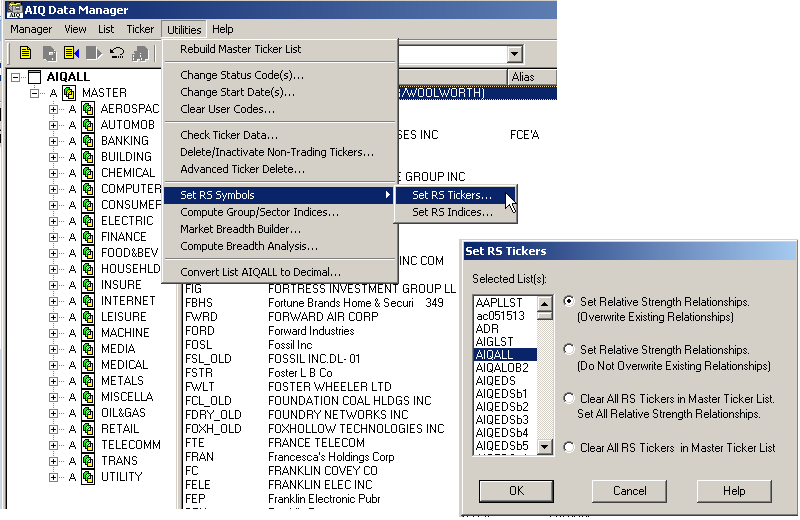FIGURE 6: AIQ DATA MANAGER. Use the AIQ Data Manager to set the RS tickers to the AIQALL list.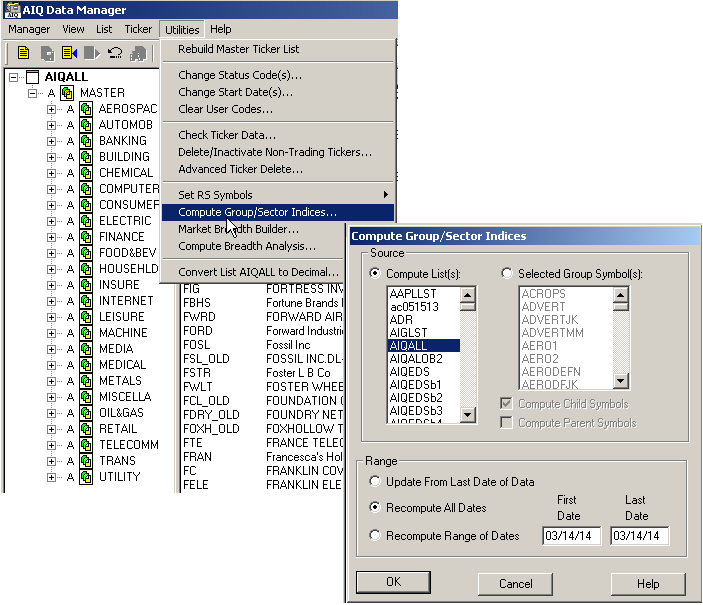FIGURE 7: AIQ DATA MANAGER. Use the AIQ Data Manager to compute the group & sector indexes for the AIQALL list.
The EDS file containing the code has the properties set to the AIQALL list. If you are building an EDS file directly from the code listing below, then be sure to set the properties to the AIQALL list.
`!A Trading Method for the Long Haul!Author: Donald W. Pendergast Jr., TASC Bonus Issue 2014!Coded by: Richard Denning 3/10/14!www.TradersEdgeSystems.com!INPUTS:trendLen is 200.rsiLen is 2.minAvgVolume is 10000.volaLen is 21.relStrLen is 80.minVolaRatio is 0.5.rsSPXmin is 1.0.rsGroupmin is 1.0.trailBars is 2.rsiBuyLvl is 5.rsiExitLvl is 95.exitLen is 18.C is [close].H is [high].L is [low].PD is {position days}.!LONG TERM MOVING AVERAGE:emaLT is expavg(C,trendLen).emaST is expavg(L,exitLen).!RSI WILDER!To convert Wilder Averaging to Exponential Averaging use this formula:!ExponentialPeriods = 2 * WilderPeriod - 1.U is C-valresult(C,1).D is valresult(C,1)-C.rsiLen1 is 2 * rsiLen - 1.AvgU is ExpAvg(iff(U>0,U,0),rsiLen1).AvgD is ExpAvg(iff(D>=0,D,0),rsiLen1).rsi is 100-(100/(1+(AvgU/AvgD))).!VOLATILITYprice1 is H.price2 is L.ratio is price1 / price2.dp is Ln(ratio).dpsqr is Ln(ratio) * Ln(ratio).totdpsqr is sum(dpsqr,volaLen).sumdp is sum(dp,volaLen).sumdpsqr is sumdp * sumdp.sumdpave is sumdpsqr / volaLen.diff is totdpsqr - sumdpave.!!use 252 for daily, or 52 for weekly belowfactor is 252 / (volaLen-1).result is sqrt(diff * factor).vola  is result * 100 .  volaAvg is expavg(vola,volaLen).volaSPXavg is tickerUDF("SPX",volaAvg).volaRatio is volaSPXavg/volaAvg.!AVERAGE VOLUMEavgVolume is expavg([volume],50).!RELATIVE STRENGTHroc is C / valresult(C,relStrLen).rocSPX is tickerUDF("SPX",roc).rocGroup is tickerUDF(rsticker(),roc).groupSymbol is tickerUDF(rsticker(),symbol()).groupName is tickerUDF(rsticker(),description()).rsSPX is roc / rocSPX.rsGroup is rocGroup / rocSPX.!SCREENING RULESVolumeRule if avgVolume > minAvgVolume.TrendRule if C > emaLT.VolaRule if volaRatio > minVolaRatio and simpleavg(H-L,10) > simpleavg(H-L,200).RelStrRule if rsSPX > rsSPXmin and rsGroup > rsGroupmin.PullbackRule if rsi < rsiBuyLvl.EnoughData if hasdatafor(trendLen+10) > trendLen.!FundamentalRule if [eps]>[eps est].Screen if EnoughDataand TrendRuleand VolaRuleand RelStrRuleand PullbackRule!and FundamentalRuleand VolumeRule.EntryTrigger if C > lowresult(H,2,1).Buy if valrule(Screen,1) and EntryTrigger.Exit if (C < lowresult(L,trailBars,1)) or (valrule(C > emaST,1) and C < emaST)orrsi > rsiExitLvl.ShowValues if EnoughData.`
—Richard Denning
for AIQ Systems

Muscle Up Those Averages (substitute article for AIQ)

Original article by Ajay Pankhania
AIQ Code by Richard Denning

The code for John Ehlers’ article is not provided for AIQ. Instead I substituted an article from the September 2013 Stocks and Commodities issue by Ajay Pankhania, “Muscle Up Those Averages”.

I thought this basic code might be useful to beginning AIQ Expert Design Studio users as it illustrates how to code multiple versions of the simple and the exponential moving averages as well as the MACD indicator. Also the simple moving average crossover system and the MACD crossover systems are coded.

EDS Code:

!MUSCLE UP THOSE AVERAGES
!Author: Ajay Pankhania, TASC Sept 2013
!Coded by: Richard Denning 11/10/2013

!INPUTS:
price is [close].
smaLen1 is 50.
smaLen2 is 200.
emaLen1 is 12.
emaLen2 is 26.
emaLen3 is 9.

!CODE FOR SIMPLE MOVING AVERAGE OF PRICE:
SMA1 is simpleavg(price,smaLen1).
SMA2 is simpleavg(price,smaLen2).

!CODE FOR CROSSOVER SYSTEM:
BuySMA if SMA1 > SMA2 and valrule(SMA1 < SMA2,1).
SellSMA if SMA1 < SMA2 and valrule(SMA1 > SMA2,1).

!CODE FOR EXPONENTIAL MOVING AVERAGES (FOR MACD):
EMA1 is expavg(price,emaLen1).
EMA2 is expavg(price,emaLen2).

!CODE FOR MACD:
Fast is EMA1 – EMA2.
Signal is expavg(Fast,emaLen3).

!CODE FOR MACD SYSTEM:
BuyMACD if Fast > Signal and valrule(Fast < Signal,1).
SellMACD if Fast < Signal and valrule(Fast > Signal,1).